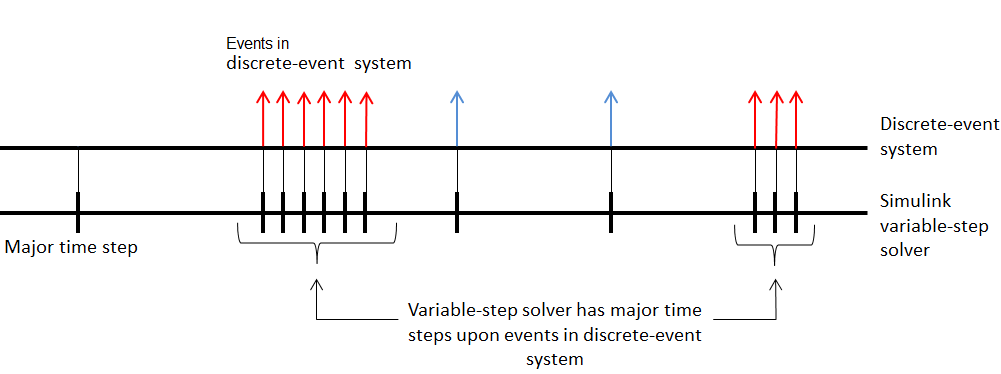## Solvers for Discrete-Event Systems

Depending on your configuration, you can use both variable-step and fixed-step solvers with discrete-event systems. To choose solver settings for your model, navigate to the Solver pane of the model Configuration Parameters dialog box.

When choosing a solver type for your model, use the following guidelines:

• If your model contains only event-based computation and excludes continuous and discrete time-based computation, choose the variable-step, discrete solver. In this case, if you select a variable-step continuous solver, the software detects that your model does not contain any blocks with continuous states (Simulink® blocks) and automatically switches the solver to `discrete (no continuous states)`. When the software makes this change, it notifies you with a message in the MATLAB® command window.

• If your discrete-event system is within a Simulink model that also contains time-based modeling, choose either a variable-step or fixed-step solver, depending on your simulation requirements. For each solver type, the following sections describe the behavior of discrete-event systems when contained within such models.

### Variable-Step Solvers for Discrete-Event Systems

If your discrete-event system is within a Simulink model that contains time-based modeling, and you choose a variable-step solver for the model, the Simulink solver has a major time step each time the discrete-event system processes events.

The following graphic illustrates the behavior of the variable-step solver when used with a discrete-event system contained within a Simulink model.### Fixed-Step Solvers for Discrete-Event Systems

If you have a discrete-event system within a Simulink model that includes time-based modeling, you can choose a fixed-step solver for the model.

When you use a fixed-step solver, the simulation still executes events in the discrete-event system at the times at which they occur. However, these events do not cause the Simulink solver to have sample hits at those times. The software insulates the discrete-event system from the time-based portions of the Simulink model.

The following graphic illustrates the behavior of the fixed-step solver when used with a discrete-event system.